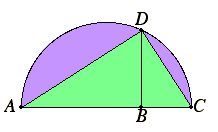# Proposition 13

To find a mean proportional to two given straight lines.

Let AB and BC be the two given straight lines.It is required to find a mean proportional to AB and BC.

I.11

Place them in a straight line, and describe the semicircle ADC on AC. Draw BD from the point B at right angles to the straight line AC, and join AD and DC.

III.31

Since the angle ADC is an angle in a semicircle, it is right.

VI.8,Cor

And, since, in the right-angled triangle ADC, BD has been drawn from the right angle perpendicular to the base, therefore BD is a mean proportional between the segments of the base, AB and BC.

Therefore a mean proportional BD has been found to the two given straight lines AB and BC.

Q.E.F.

## Guide

This construction of the mean proportional was used before in II.4 to find a square equal to a given rectangle. By proposition VI.17 coming up, the two constructions are equivalent. That is the mean proportional between two lines is the side of a square equal to the rectangle contained by the two lines. Algebraically, a : x = x : b if and only if ab = x2. Thus, x is the square root of ab. This mean proportional between a and b is also called the geometric mean of a and b.

When b is taken to have unit length, this construction gives the construction for the square root of a.

#### Use of this proposition

This construction is used in the proofs of propositions VI.25, X.27, and X.28.